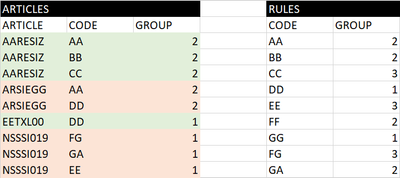cancel
Showing results for
Did you mean:Helper II

## Get max value from related table list

Hello all,

In a data quality control project, I want to compare two groups, one entered by a user and the other defined by a rule.Articles[CODE] is related many-to-one with Rules[CODE], because Articles can have several codes.

Each code is related to a group. Group values are either 1, 2 or 3.

There is a hierarchy between groups ; if article XX9EODS has two codes : AAA -> group = 1 and BBB -> group = 2 then Articles[GROUP] will be 1. Articles can only have one group.

With these information, I imagine to get all codes related to one article, then compare it to the codes in the Rules table, then get the min of the selected groups in the Rules table and finally compare this min to the one related to the article and return 1 if correct and 0 if not. And do this for each article.

Here is one example:Should I use LOOKUPVALUES(), SUMMARIZECOLUMNS(), etc. ?

I think I have not enough DAX knowledge to achieve this. Could you help me?

Thanks!

3 REPLIES 3Super User

Please always show your sample data in text-tabular format in addition to (or instead of) the screen captures. A screen cap doesn't allow people to readily copy the data and run a quick test and thus decreases the likelihood of your question being answered. Just use 'Copy table' in Power BI and paste it here. Or, ideally, share the pbix (beware of confidential data).

Try the following:

1. Delete the relationship between the two tables

2. Place Article, Code and Group of the Articles table in a table visual (all set no "Don't summarize", so that it shows exactly as in your pic above)

3. Create this measure and place it in the visual:

``````Measure =
VAR articleCodes_ =
CALCULATETABLE (
DISTINCT ( Articles[Code] ),
ALLEXCEPT ( Articles, Articles[Code] )
)
VAR correctCode_ =
MINX ( FILTER ( Rules, Rules[Code] IN articleCodes_ ), [Group] )
VAR currentCode_ =
SELECTEDVALUE ( Articles[Code] )
RETURN
IF ( currentCode_ = correctCode_, 1, 0 )``````

All this can also be done in the Articles table itself, by adding a calculated column. And it can be done as well in Power Query, which would probably be the best option

Please mark the question solved when done and consider giving a thumbs up if posts are helpful.

Contact me privately for support with any larger-scale BI needs, tutoring, etc.

CheersHelper II

Example added, with correct group values in green and wrong group values in red.Super User

Hi @Grob2

Can you show an example based on data to help clarify the requierement please?

Please mark the question solved when done and consider giving kudos if posts are helpful.

Contact me privately for support with any larger-scale BI needs, tutoring, etc.

Cheers